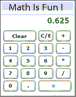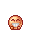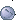# How to convert a Fraction into a decimal?

+11 votes
212 views
asked Apr 16, 2013 in Math

I started 5th grade 3 months ago, and we were supposed to learn this earlier, but I was away. I just don't get it!commented Jun 17, 2019 by vip

U have to no your place value than it will get better. Do you no how to do place valau?I now math is boring sometimes.## 3 Answers

+2 votes
answered Apr 16, 2013 by (41,980 points)

Best answer
Take your fraction. Pretend it is 3/4. This fraction is like a division problem in itself. Basically it is 3 divided by 4. If you use your calculator, divide 3 by 4. You get 0.75, right?

Basically if you have a calculator you can do this!

Hope this helps!!!
+1 vote
answered Apr 17, 2013 by (32,950 points)
I havent learned how to yet but i will in my next math chapter
+1 vote
answered Apr 17, 2013 by (32,330 points)

The way you convert a Fraction into a decimal is by turning the fraction into a fraction with a denominator of 100. Then you write a decimal point with the the numerator there behind it. If the fraction is one digit number you put the decimal point and write that one digit number. If the numerator is a two digit number you put that number behind the decimal.

I understand what you feel, sometimes I don't get things either, but you just have to try it at least.

Hope this helps!